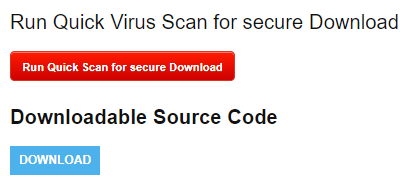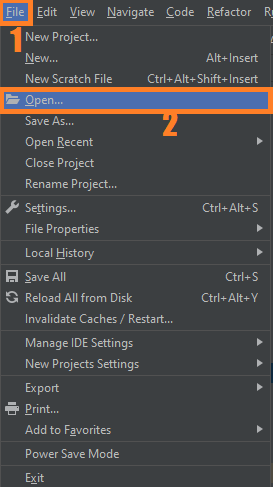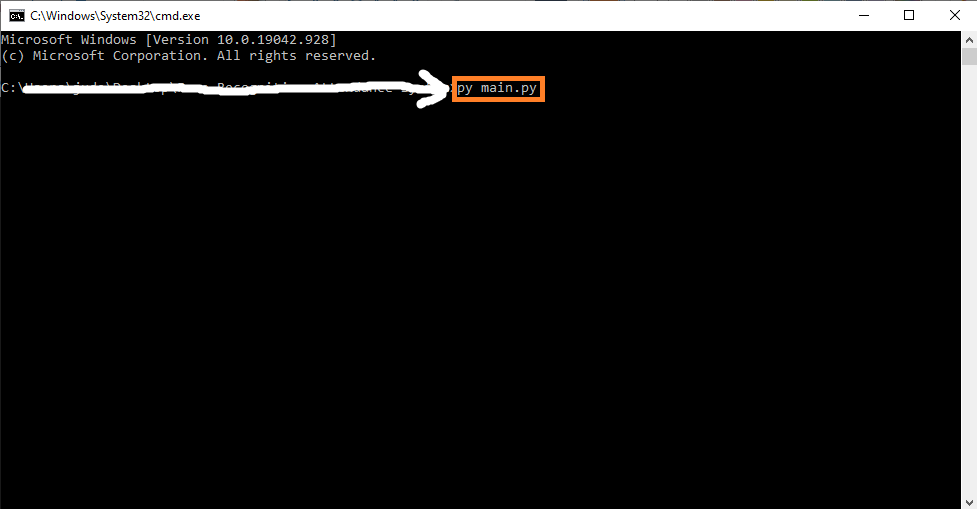# Real-Time Object Detection OpenCV Python With Source Code

## Real Time Object Detection OpenCV Python With Source Code

The Real-Time Object Detection OpenCV Python was developed using Python OpenCV, This opencv realtime object detection script is a simple experimental tool to detect common objects (COCO) easily with your built-in webcam.

It uses opencv’s readNet method and uses the external yolov3-tiny model (which can be upgraded to the full sized model).

Opencv’s readNet method only runs on CPU (and not GPU), is very intensive, and therefore, it will be not be optimal for big AI projects.

A Object Detection OpenCV Python implements an image and video object detection classifier using pretrained yolov3 models.

The yolov3 models are taken from the official yolov3 paper which was released in 2018. The yolov3 implementation is from darknet. Also, this project implements an option to perform classification real-time using the webcam.

## Project Information’s

By the way if you are new to python programming and you don’t know what would be the the Python IDE to use, I have here a list of Best Python IDE for Windows, Linux, Mac OS that will suit for you. I also have here How to Download and Install Latest Version of Python on Windows.

To start executing Real-Time Object Detection OpenCV Python With Source Code, make sure that you have installed Python 3.9 and PyCharm in your computer.

## Steps On How To Run The Real Time Object Detection OpenCV Python With Source Code

Time needed: 5 minutes.

These are the steps on how to run Real-Time Object Detection OpenCV Python With Source Code

First, download the given source code below and unzip the source code.• Step 2: Import the project to your PyCharm IDE.• Step 3: Run the project.

last, run the project with the command “py main.py”## Installed Libraries

```import cv2
import numpy as np
import time```

## Complete Source Code

```import cv2
import numpy as np
import time

classes = []
with open("./configuration/coco.names", "r") as f:
classes = [line.strip() for line in f.readlines()]
layer_names = net.getLayerNames()
output_layers = [layer_names[i - 1] for i in net.getUnconnectedOutLayers()]
colors = np.random.uniform(0, 255, size=(len(classes), 3))

cap = cv2.VideoCapture(0)
font = cv2.FONT_HERSHEY_SIMPLEX
starting_time = time.time()
frame_id = 0

while True:
frame_id += 1
height, width, channels = frame.shape

# Detecting objects
blob = cv2.dnn.blobFromImage(frame, 0.00392, (416, 416), (0, 0, 0), True, crop=False)
net.setInput(blob)
outs = net.forward(output_layers)

# Visualising data
class_ids = []
confidences = []
boxes = []
for out in outs:
for detection in out:
scores = detection[5:]
class_id = np.argmax(scores)
confidence = scores[class_id]
if confidence > 0.1:
# Object detected
center_x = int(detection * width)
center_y = int(detection * height)
w = int(detection * width)
h = int(detection * height)

# Rectangle coordinates
x = int(center_x - w / 2)
y = int(center_y - h / 2)

boxes.append([x, y, w, h])
confidences.append(float(confidence))
class_ids.append(class_id)

indexes = cv2.dnn.NMSBoxes(boxes, confidences, 0.8, 0.3)

for i in range(len(boxes)):
if i in indexes:
x, y, w, h = boxes[i]
label = str(classes[class_ids[i]])
confidence = confidences[i]
color = colors[class_ids[i]]
cv2.rectangle(frame, (x, y), (x + w, y + h), color, 2)
cv2.putText(frame, label + " " + str(round(confidence, 2)), (x, y + 30), font, 3, color, 3)

elapsed_time = time.time() - starting_time
fps = frame_id / elapsed_time
cv2.putText(frame, "FPS: " + str(round(fps, 2)), (40, 670), font, .7, (0, 255, 255), 1)
cv2.putText(frame, "press [esc] to exit", (40, 690), font, .45, (0, 255, 255), 1)
cv2.imshow("Image", frame)
key = cv2.waitKey(1)
if key == 27:
print("[button pressed] ///// [esc].")
print("[feedback] ///// Videocapturing succesfully stopped")
break

cap.release()
cv2.destroyAllWindows()```

## Summary

This project implements an image and video object detection classifier using pretrained yolov3 models. The yolov3 models are taken from the official yolov3 paper which was released in 2018. The yolov3 implementation is from darknet. Also, this project implements an option to perform classification real-time using the webcam.

## Inquiries

1.•